# 请保护好自己，开发员！

8 四月 2016, 16:26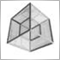0
2 929

### 保护字符串常量

```//+------------------------------------------------------------------+
//|                                                  Protect-001.mq4 |
//|                                         http://forextools.com.ua |
//+------------------------------------------------------------------+

int start()
{
return(0);
}
```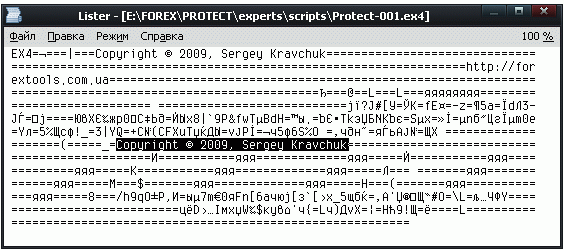```//+------------------------------------------------------------------+
//|                                                  Protect-002.mq4 |
//|                                         http://forextools.com.ua |
//+------------------------------------------------------------------+

#property show_inputs

extern string prefix = "char";
extern string text   = "input your text for encoding";
string rez           = ""; // here we will assemble the result

int start()
{
// enter the original text to see what this string is
rez = "/* " + text + " */ ";

for (int i = 0; i < StringLen(text); i++)
rez = rez + prefix + "[" + StringGetChar(text, i) + "]+";

// cut the last '+' character and print string to the log
Print(StringSubstr(rez, 0, StringLen(rez)-1));
}
```

```//+------------------------------------------------------------------+
//|                                                  Protect-003.mq4 |
//|                                         http://forextools.com.ua |
//+------------------------------------------------------------------+

int start()
{
string char; int i;

for (i = 0; i < 256; i++) char[i] = CharToStr(i);

Comment
(
char  + char + char + char + char + char + char +
char + char + char  + char + char  + char  + char  +
char  + char  + char  + char  + char  + char + char +
char + char + char + char  + char  + char + char  +
char + char  + char + char + char
);
return(0);
}
```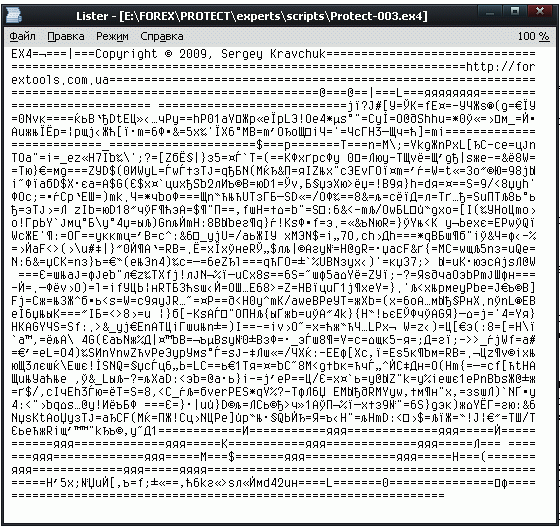### 防止未经授权使用

```//+------------------------------------------------------------------+
//|                                                  Protect-004.mq4 |
//|                                         http://forextools.com.ua |
//+------------------------------------------------------------------+

int start()
{
string char; int i;

for (i = 0; i < 256; i++) char[i] = CharToStr(i);
// Account number, on which expert is allowed to work
int    AllowedAccountNo = StrToInteger(/* 49153 */ char+char+char+char+char);
string AllowedServer = /* UWC-Demo.com */ char+char+char+char+char+
char+char+char+char+char+char+char;

if (AccountNumber() != AllowedAccountNo || AccountServer() != AllowedServer)
{
Print("You don't have permission to use this script!");
return(1);
}

Print("You can use this script!");
}
```

### 通过时间来保护

```//+------------------------------------------------------------------+
//|                                                  Protect-005.mq4 |
//|                                         http://forextools.com.ua |
//+------------------------------------------------------------------+

int start()
{
string char; int i;

for (i = 0; i < 256; i++) char[i] = CharToStr(i);

// Date, until which the expert is allowed to work
int LastAllowedDate = StrToTime(
/* 2009.09.11 23:59:00 */
char+char+char+char+char+char+char+char+char+
char+char+char+char+char+char+char+char+char+char

);

if (TimeCurrent() >= LastAllowedDate)
{
Print("Demo period has expired " + TimeToStr(LastAllowedDate,TIME_DATE|TIME_SECONDS));
return(1);
}

Print("You can work until "+ TimeToStr(LastAllowedDate,TIME_DATE|TIME_SECONDS));
}
```

### 反向编译保护方法

MQL4 编译器开发人员正疲于应对这个问题。 如何应对的细节当然不会公之于众。 但遗憾的是，源代码往往可以恢复。 尽管其文本很难读取：所有识别器而非有意义的名称会机械性地创建名称。 这使回顾和重建算法的过程大为复杂化。 在拥有多个函数的大文件内，这会成为几乎无法解决的问题。 但是，对于小型 expert 而言，它可以重新恢复至接近初始状态。

```//+------------------------------------------------------------------+
//|                                                  Protect-006.mq4 |
//|                                         http://forextools.com.ua |
//+------------------------------------------------------------------+

int start()
{
string char; int i;

for (i = 0; i < 256; i++) char[i] = CharToStr(i);

// Date, until which the expert is allowed to work
int LastAllowedDate = StrToTime(
/* 2009.09.11 23:59:00 */
char+char+char+char+char+char+char+char+char+
char+char+char+char+char+char+char+char+char+char

);

if (IsDemoMode(LastAllowedDate))
{
Print("Demo period has expired " + TimeToStr(LastAllowedDate,TIME_DATE|TIME_SECONDS));
return(1);
}

Print("You can work until "+ TimeToStr(LastAllowedDate,TIME_DATE|TIME_SECONDS));
}

bool IsDemoMode(int LastAllowedDate)
{
if (TimeCurrent() >= LastAllowedDate) return(true); else return(false);
}
```

```#property copyright "Copyright © 2009, Sergey Kravchuk"

int start() {
string lsa_0;
for (int l_index_4 = 0; l_index_4 < 256; l_index_4++) lsa_0[l_index_4] = CharToStr(l_index_4);
int l_str2time_8 = StrToTime(lsa_0 + lsa_0 + lsa_0 + lsa_0 + lsa_0 +
lsa_0 + lsa_0 + lsa_0 + lsa_0 + lsa_0 + lsa_0 + lsa_0 +
lsa_0 + lsa_0 + lsa_0 + lsa_0 + lsa_0 + lsa_0 + lsa_0);
if (IsDemoMode(l_str2time_8)) {
Print("Demo period has expired " + TimeToStr(l_str2time_8, TIME_DATE|TIME_SECONDS));
return (1);
}
Print("You can work until " + TimeToStr(l_str2time_8, TIME_DATE|TIME_SECONDS));
return (0);
}

bool IsDemoMode(int ai_0) {
if (TimeCurrent() >= ai_0) return (TRUE);
return (FALSE);
}
```

### MQLEnigma - MQ4 源代码的编码器

MQLEnigma - 是一款控制台应用程序。 该程序有强制参数 - 用于编码的文件名。 此后，将对文本进行以下操作：

2. 从得出的总文本中删除所有注释（不要遇到类似 // int i=0 的东西）。

3. 将已清除的文本分成两部分：函数主体（它们被删除并单独分析），和所有外部变量和 #define（以及函数声明）。

4. 从外部文本块中移除所有导入函数的描述。 它们由外部命名，无法被编码。

5. 在两个文本块（外部和内部）中找到常用类型的描述，如 int, string 等等。 从这些类型中删除变量初始化和数组维度。

6. 从 #define 部分删除 #define 关键字和参数设置，从而仅留下已定义的名称。 同时删除已保留的关键字（即你不会不小心将洋红色名称更换为一些无用资料）。

7. 因此，仅留下了变量名称、内部函数和已声明的常量。 这些名称将收入常用列表内，复制项目将从该列表中移除（如 int i=0; 这样的描述可在部分函数内发生）。 创建用于更换的“无用资料”变量名称并确定找到的识别器长度，从而从最长的那个开始执行更换。 否则，如果出现像 str 和 str2str 这样的两个变量，如果先更换两个 str “部分”的话，则最长的 str2str 的名称会出错。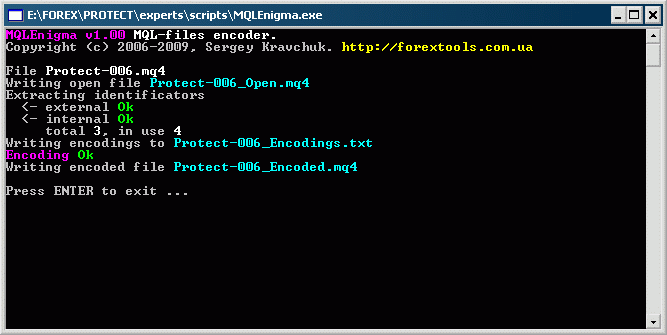`Print(" LotSize = " + DoubleToStr(LotSize,2));`

```21      char             2114319876214748364748749
6       i                1596751872214748364643597
1       IsDemoMode       158651616521474836452710
5       LastAllowedDate  3650920921474836448123 ```

```#property copyright "Copyright © 2009, Sergey Kravchuk"
int start()
{
string  2114319876214748364748749 ; int  1596751872214748364643597 ;
for ( 1596751872214748364643597  = 0;  1596751872214748364643597  < 256;  1596751872214748364643597 ++)
2114319876214748364748749 [ 1596751872214748364643597 ] = CharToStr( 1596751872214748364643597 );
int  3650920921474836448123  = StrToTime(
2114319876214748364748749 + 2114319876214748364748749 + 2114319876214748364748749 +
2114319876214748364748749 + 2114319876214748364748749 + 2114319876214748364748749 +
2114319876214748364748749 + 2114319876214748364748749 + 2114319876214748364748749 +
2114319876214748364748749 + 2114319876214748364748749 + 2114319876214748364748749 +
2114319876214748364748749 + 2114319876214748364748749 + 2114319876214748364748749 +
2114319876214748364748749 + 2114319876214748364748749 + 2114319876214748364748749 +
2114319876214748364748749 
);
if ( 158651616521474836452710 ( 3650920921474836448123 ))
{
Print("Demo period has expired " + TimeToStr( 3650920921474836448123 ,TIME_DATE|TIME_SECONDS));
return(1);
}
Print("You can work until "+ TimeToStr( 3650920921474836448123 ,TIME_DATE|TIME_SECONDS));
}
bool  158651616521474836452710 (int  3650920921474836448123 )
{
if (TimeCurrent() >=  3650920921474836448123 ) return(true); else return(false);
}
```

```#property copyright "Copyright © 2009, Sergey Kravchuk"

int start() {
string lsa_0;
for (int l_index_4 = 0; l_index_4 < 256; l_index_4++) lsa_0[l_index_4] = CharToStr(l_index_4);
int l_str2time_8 = StrToTime(lsa_0 + lsa_0 + lsa_0 + lsa_0 + lsa_0 +
lsa_0 + lsa_0 + lsa_0 + lsa_0 + lsa_0 + lsa_0 + lsa_0 +
lsa_0 + lsa_0 + lsa_0 + lsa_0 + lsa_0 + lsa_0 + lsa_0);
if (f0_1276(l_str2time_8)) {
Print("Demo period has expired " + TimeToStr(l_str2time_8, TIME_DATE|TIME_SECONDS));
return (1);
}
Print("You can work until " + TimeToStr(l_str2time_8, TIME_DATE|TIME_SECONDS));
return (0);
}

bool f0_1276(int ai_0) {
if (TimeCurrent() >= ai_0) return (TRUE);
return (FALSE);
}
```

### 总结

MQLEnigma 的源代码 可能包含部分在调试期间未出现在我文本中的逻辑错误。 如果你找到这些错误，请告诉我以便我进行修改并更新本文随附文件。 如果你了解其他可以公开发表而不会被黑客利用的保护方法，请留下你的评论。 我和我们 MQL4 社区内的其他成员都会对你的贡献深表感激。

files_en.zip (29.18 KB)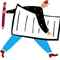Meta 交易者持仓报告 - 在 MetaTrader 4 中进行美国商品期货交易委员会报告分析的新领域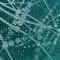FANN2MQL 神经网络教学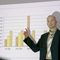金融证券的叠加和干扰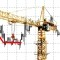交易者的工具箱: 设计指标